×

×

UA / Science / EN 101 / millikan's "oil drop" apparatus allowed for the determination of which

# millikan's "oil drop" apparatus allowed for the determination of which Description

##### Description: 13 pages of information This study guide covers Chapters 1 through 3, which will be tested on the first exam combines information from in class lectures, the textbook, and internet research
14 Pages 5 Views 12 Unlocks
Reviews

mcperron (Rating: )

Chapter 1

## States of matter● Elements, compounds, and mixtures

Elements are substances that cannot be chemically broken down into simpler  substances. Compounds are substances composed of two or more elements in fixed, definite proportions. Mixtures are composed of more than one particle and can be  broken down into simpler substances; they are either homogenous or  heterogeneous, depending on how uniformly the particles are distributed.

● What is a molecule?

Atoms bound together in a specific geometrical arrangement. They are  extremely small and their properties are determined by the arrangement of the  atoms.

● States of matter

This is determined by the relative position of particles and how strongly they  interact with one another. States include: solid, liquid, and gas. Solid matter has  strong attraction and are packed closely together; which is why solids have a fixed  volume and shape. Liquid matter is packed closely together too, but has a weaker  attraction than that of solid matter; liquids have a fixed volume but not shape.  Gaseous matter has very weak attraction and do not clump together; thus gaseous  matter does not have a fixed volume or shape.

## Laws, theories, observations, and measurements● Laws, theories, observations, and measurements

A scientific law is a statement based on repeated experimental observations  that describes how nature behaves. A law always applies under the same  conditions, and implies that there is a causal relationship involving its elements. A  scientific theory is a well-supported explanation of some aspect of the natural world, based on a body of facts that have been repeatedly confirmed through observation  and experiment (a theory is not a guess by any means). Observations involve  gathering information through the senses and are often done so quantitatively with  measurements (numbers).  We also discuss several other topics like gabrielle floyd
Don't forget about the age old question of film 2700 gsu exam 1

● Law of conservation of mass

The law of conservation of mass states that mass in an isolated system is  neither created nor destroyed during chemical or physical reactions Therefore, the  mass of the products in a chemical reaction must equal the mass of the reactants

## Law of conservation of mass● Law of definite proportions

The law of definite proportions states that a chemical compound always  contains exactly the same proportion of elements by mass. If you want to learn more check out thermodynamics exam 1

● Law of multiple proportions

The law of multiple proportions states that elements combine in whole  number ratios.

● Dalton’s Atomic theory

- each element is composed of tiny, indestructible atoms

- all atoms of the same element have identical mass and properties to each  other, but different from the atoms of other elements

- atoms combine in simple, whole number ratios to form compounds

- atoms of one element cannot change into atoms of another element. In  chemical reactions, they only change the way they are bound to other atoms

● J.J. Thomson’s cathode ray experiment

Thompson applied a high electrical voltage between two electrodes at either  end of a partially evacuated glass tube (a cathode ray tube). He found that the  beam of particles (cathode rays) traveled from the negatively charged electrode (cathode) to the positively charged electrode (anode). He also found that they are  negatively charged. Electrical charge is what results in attractive and repulsive  forces -called electrostatic forces- between the charged particles.  We also discuss several other topics like Chapter 1: Thinking Critically with Psychological Science WHAT IS PSYCHOLOGY?

He also found the charge to mass ratio of the particles by deflecting them  using electric and magnetic fields and finding at which measurement they canceled, leaving the beam undeflected. The value is -1.76 x 108 coulombs per gram (this is  2000 times lighter than Hydrogen, the lightest known atom). He discovered the  electron.

● Millikan’s oil drop experiment

Milikan sprayed oil into an apparatus. In the top half of the apparatus, he hit  the air with ionizing radiation which allowed the drops to gain electrons. In the  bottom half of the apparatus, he applied an electric voltage. The electric field  produced by this voltage would act on the charged oil drops and the  electromagnetic force would just balance the force of gravity on a drop, and the  drop would hang suspended in mid-air. Using the strength of the electric field and  the mass of the drops themselves, he calculated the charge of each drop.

He found that the charge of each drop must be a whole number multiple of  the electrons charge. The charge of a single atom: -1.60 x 10-19 C. This number,  combined with Thompson’s mass to charge ratio, gave the mass of a single  electron: 9.10 x 10-28 g.

The charge of an electron determines how strongly it holds its bonds. ● Rutherford’s gold foil experiment

Rutherford shot a beam of alpha particles at a sheet of gold foil, a few of  the particles were deflected (this was unexpected). He concluded that a tiny, dense  nucleus was causing the deflections. If you want to learn more check out are high speed central networks to which multiple smaller networks connect

He found that an atom was much more than just empty space and  scattered electrons and that it must have a positively charged center that contains  most of its mass (the nucleus).

● Sub atomic particles and their properties

Subatomic particles include protons, neutrons, and electrons. Protons carry a  positive charge and a mass of 1.00727 amu; the number of protons defines an  element. Neutrons carry a neutral charge (no charge) and a mass of 1.00866 amu.  Electrons carry a negative charge and a mass of 0.00055 amu.

● Atomic symbols

Atomic symbols illustrate the mass number (upper left corner), the atomic  number (bottom left corner), and the symbol (large letter/s) of an element.

● isotopes

Isotopes are atoms with the same number of protons but different number of  neutrons.

Ex. Carbon-13 has 6 protons but 7 neutrons. We also discuss several other topics like adrian michael pitt

● atomic masses

○ convert to g/mol

Step 1: Determine how many grams are given in the problem.

Step 2: Calculate the molar mass of the substance.

Step 3: Divide grams given by molar mass.

Ex. Convert 25.0 grams of KMnO4 to moles

Step 1: 25.0 g

Step 2: K (39.1) + Mn (54.9) + O (16 x 4)

Molar mass of KMnO4 is 158.034 grams/mole

Step 3: 25 / 158 = 0.158 mol

○ find an atomic mass from an isotopic mass, or vice versa

Ex. In a sample of 400 lithium atoms, it is found that 30 atoms are lithium-6  (6.015 g/mol) and 370 atoms are lithium-7 (7.016 g/mol). Calculate the average  atomic mass of lithium

Step 1: Calculate the percent abundance for each isotope:

Li-6: 30/400 = 0.075

Li-7: 370/400 = 0.925

Step 2: Calculate the average atomic weight:

x = (6.015) (0.075) + (7.016) (0.925)

x = 6.94 g/mol

Chapter 2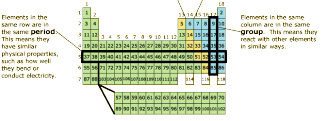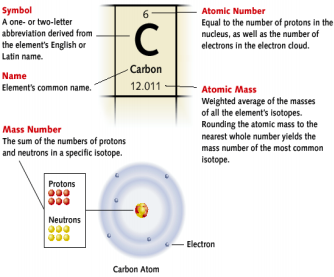● Significant figures

Rules

Examples

Zeros appearing between nonzero  digits are significant

Zeros appearing in front of nonzero  digits are not significant

Zeros at the end of a number and to the right of a decimal are significant

Zeros at the end of a number but to the left of a decimal may or may not be  significant. If such a zero has been  measured, or is the first estimated digit, it is significant. On the other hand, if  the zero has not been measured or  estimated but is just a placeholder, it is  not significant. A decimal placed after  the zeros indicates that they are

40.7 L has three sig figs

87 009 km has five sig figs

0.095 987 m has five sig figs

0.0009 kg has one sig fig

85.00 g has four sig figs

9.000 000 000 mm has 10 sig figs

2000 m may contain from one to four  sig figs, depending on how many zeros  are placeholders. For measurements  given in this text, assume that 2000 has one sig fig.

2000. m contains four sig figs, indicated by the presence of the decimal point

significant.

Scientific notation - All digits expressed  before the exponential term are  significant.

5.060 x 10-3 m has four sig figs. 9.00 x 102 g has three sig figs.

● Precision vs. accuracy

Accuracy is how close the measured value is to the actual value. For  example, if in a lab you obtain a weight of 3.2 kg for a substance, but the actual  weight is 10 kg, then your measurement is not accurate because it is not close to  the correct value.

Precision is how close the measurements are to each other. For example, if  you weigh a substance five times, and get 3.2 kg each time, then your  measurement is very precise.

● Density

Density of a substance is the ratio of its mass (m) to its volume (V). Its units  are g/cm3

Ex. What is the density of a solid substance that has a mass of 22.5 g and a  volume of 2.238 cm3?

22.5 g

2.38cm3 = 9.45 g/cm3

● Energy

d=

m

V =

Energy is the capacity to do work; work is the action of a force through a  distance. Total energy is the sum of an objects kinetic energy. Kinetic energy is  associated with motion (ex. A weight falling through space and accelerating).  Potential energy is associated with the objects position or composition (ex. The  weight sits on a table a few meters above the ground- it has potential energy due to its position within earths gravitational field). Thermal energy is a type of kinetic  energy associated with the temperature of an object (ex. If the weight hits the  ground, its kinetic energy is converted to thermal when it transfers to the atoms  that make up the ground).

m2

The SI unit of energy is kg x m2s2 (Joule). 1 kg

s2 = 1 J

Energy is conserved in any process (law of conservation of energy – energy is neither created nor destroyed) but can be transferred between a system and its  surroundings. If the system loses energy, the process is exothermic; if the system  gains energy, the process is endothermic.

● Unit Conversion

Ex. Convert 12.5 inches to cm 1∈¿

Unit we are

converting to

12.5 in x

2.54 cm

¿ = 31.8 cm

Unit we are converting

from

Ex. Convert 1.8 quarts to centimeters Steps: qt  L  mL  cm3

1.057 qt x 1mL

10−3L x 1 cm3

1mL = 1.70293 x 103 cm3  1.7 x

103 cm3

1L

1.8 qt x

Ex. Calculate the displacement (the total volume of cylinders through which  the piston moves) of a 5.70 L automobile engine in in3

Steps: L  mL  cm3  in3

1∈¿

5.70 L x

1mL

10−3L x

1 cm3

1mL x

¿¿3

¿¿¿ = 347.835 in3  348 in3

● Orders of magnitude and scientific notation

In the order of magnitude estimation technique, we focus only on the  exponential part of numbers written in scientific notation. If the decimal is less than  5, drop it (ex. 4.36 x 105  105). If the decimal is 5 or more, round up to 10 and  rewrite the power of 10 (ex. 5.982 x 107  10 x 107 108).

● The mole

A mole is the amount of material containing 6.02214 x 1023 particles  (Avogadro’s number). Moles are unit less, so we usually refer to the number of  moles of a substance as the amount of a substance (ex. 1 mol of marbles = 6.02214 x 1024 marbles).

The mole gives us the relationship between mass and number of atoms.  1 mol atoms

Conversion factors:

6.02214 x1023 atoms or 6.02214 x1023 atoms 1 mol atoms

Ex. Calculate the number of copper atoms in 2.45 mol of copper

2. 45 mol Cu x 6.02214 x1023Cu atoms

1molCu = 1.48 x 1024 Cu atoms

Ex. Calculate the amount of carbon (in moles) in a 0.0265 g pencil

Convert between mass and moles using the elements molar  mass

1mol C

0.0265 g C x

12.01 gC = 2.21 x 10-3 mol C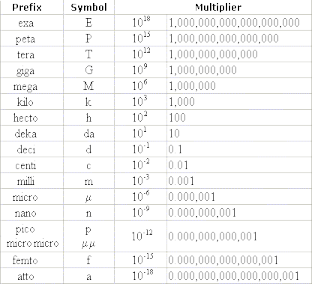● The following metric prefixes: T, G, M, k, c, m, μ, n

Chapter 3

● Properties of light waves

Amplitude – the vertical length of a crest or trough; determines  intensity/brightness

The greater the amplitude, the greater the intensity (brighter  color)

Wavelength () – the distance between two analogous points (i.e. crests);  determines color

Frequency (ν) – the number of cycles that pass through a point in a period of  time (unit = Hertz)

The frequency of a wave is directly proportional to its speed

The frequency of a wave is inversely proportional to its

wavelength

v=cλ c = speed of light = 3.00 x 108 m/s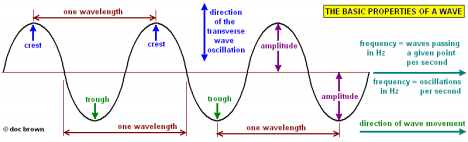Interference – the way waves interact

If two waves of equal

amplitude are in phase (align  with overlapping crests)

interact, it results in wave with twice the amplitude.

If two waves of equal

amplitude are out of phase  (the crest of one aligns with  the trough of another)

interact, their waves cancel  out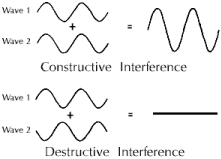Diffraction – when a wave bends around an obstacle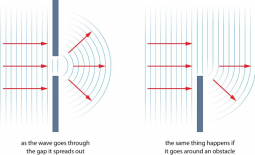● Wave particle duality

Light and matter act as both waves and particles.

The wave nature is seen most clearly through diffraction.

The wave and particle nature of electrons are said to be complementary. This means they exclude one another – the more we know about one, the less we  know about the other (which one we observe depends on the experiment we  perform)

● The Photoelectron Effect

The photoelectron effect is the observation that metals emit electrons when  light shines on them as long as the energy of the photon is greater than the energy  with which the is bound to the metal.

Low frequency (long wavelength) light does no eject electrons from metal. High Frequency (short wavelength) light does eject electrons form metal.

Albert Einstein proposed that light energy came in packets (photons) and the  amount of energy depended on the frequency. This meant light was not a  continuous wave, but a series of photons with a certain amount of energy.

E = hv where h = Planck’s constant and v = frequency

But since v=cλ , it can also be expressed as

hc

E=

λ where h = Planck’s constant, c = speed of light, and  = wavelength

Ex. A nitrogen gas laser pulse with a pulse wavelength of 337 nm contains  3.83 mJ of energy. How many photons does it contain?

10−9

 = 337 nm x

so units cancel

1nm = 3.37 x 10-7 m Convert nm to meters

λ = (6.626 x 10−34 kg m2s−2)(3.00 x108m s−1)

3.37 x 10−7m = 5.8985 x

10-19 J

hc

Ephoton =

3.83 mJ x

10−3J

1 J = 3.83 x 10-3 J Convert mJ to J

# of photons =

photons

● Atomic Spectra

Energy of the laser energy of a photon =

3.83 x 10−3J

5.8985 x 10−19 J = 6.49 x 10-5

When an atom absorbs energy, it reemits the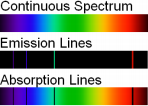energy as light at various wavelengths.

By passing the light emitted by a single atom

through a prism, we can separate the light into its different

wavelengths. This results in an emission spectrum- a

series of bright lines that is unique to each element.

A white light spectrum is continuous and displays light of all wavelengths. An  emission spectrum is not continuous and only displays bright lines of light at certain wavelengths.

● The Bohr model

Bohr’s model depicted electrons traveling around in orbits  around the nucleus at specific, fixed distances. The energy at each orbit is also fixed unless the electron jumps from one orbit to  another.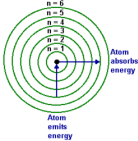His model explained that electrons could jump from

one orbit to another only by emitting or absorbing energy in  fixed amounts. If an electron jumps one orbit closer to the

nucleus, it must emit energy equal to the difference of the energies of the two orbits. But if the electron jumps to a larger orbit, it must absorb energy equal to the difference in orbits.

His model was later replaced by one that completely incorporates the wave  nature of electrons.

● the de Broglie wavelength

λ=hmv Relationship between wavelength (), mass (m), and velocity (v)

An electrons wavelength is related to its kinetic energy. Its kinetic  energy is determined by its momentum, which is the electrons mass times its  velocity. An electrons wavelength is inversely proportional to its momentum

More motion more kinetic energy  smaller wavelength

Ex. Calculate the wavelength of an electron traveling with speed of 2.65 x 106 m/s

m= 9.11 x 10-31 kg v= 2.65 x 106 m/s h= 6.626 x 10-34 kg m2s-2

λ=6.626 x 10−34 kg m2s−2

(9.11 x 10−31 kg)(2.65 x106m/s) = 2.74 x 10-10 m

● Probability distributions in matter

Probability distribution maps show where an electron is likely to be found  under certain conditions. The path an electron will take is indeterminate (we don’t  know where it will land) but after observing where numerous electrons land in  certain conditions, a statistical pattern can be made. However, orbitals are used to  describe the electrons position, not location. Therefore, orbitals are a probability  density distribution map of the electron, or the path the electron will likely take.

● The Schrödinger equation

It is used to find the wave functions and allotted energy levels (orbitals) of an  electron

HΨ = EΨ H = set of operations representing the atoms total energy  (potential and kinetic)

E = the actual energy of the electron

Ψ = the wave function that describes the wave nature of the

electron

Ψ2 = represents the orbital

● The wave functions of hydrogen

○ Quantum numbers

1) The Principal Quantum Number (n) – an integer (1, 2, 3, …) that  determines the overall size and energy of an orbital. Orbitals with higher n values have more energy and the space between energy levels decreases. The energy is negative because it is lowered according to its interaction  with the nucleus.

Ex. Determine the energy of hydrogen electron in the second orbital 1

En = -2.18 x 10-18 J (

22 ) = -5.45 x 10-19 J

1

2.18 x 10-18 J = Rydberg’s Constant for Hydrogen (RH) orbital

n2→n=

2) The Angular Momentum Quantum Number (l) – integer that determines  the shape of the orbital. The range of possible values of l depending on n.  If n = 1, l = 1 -1 = 0. If n=2, l= 2-1 = 0 or 1. To avoid confusion, values of l are assigned letters: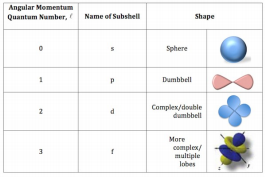3) The Magnetic Quantum Number (m1) – an integer that specifies the

orientation of the orbital. Its values range from the negative of the angular momentum quantum number to the positive of the angular momentum

quantum number. If l = 2, then m1 = -2, -1, 0, +1, +2)

4) The Spin Quantum Number (ms) – specifies the orientation of the spin of  the electron. The electrons spin is a fundamental property of the electron  and all electrons have the same amount of spin, but they can spin

different ways. Either up (ms = +1/2) or down ((ms= -1/2)

Same value of n = same

principal level (or shell).

Same value of n and l = same  sublevel (or subshell)

Number of sublevels = n

Number of orbitals in a sublevel = 2l +1

Number of orbitals in a level =  n2

○ orbitals and their shapes

The shape that encompasses the volume where the electron is likely to be found

probability

Ψ2 = probability density=

unit volume

Probability density = probability per unit volume of finding the electron at a point

s Orbitals (l = 0)

Lowest energy orbital

Spherically symmetrical

A three-dimensional plot of Ψ2

p Orbitals (l = 1)

Each principal level with n =2 or greater has three p orbitals (ml = -1,  0, +1)

p orbitals have two lobes of electron density on either side of the  nucleus and a node in the middle

The three p orbitals differ only in their orientation

They are orthogonal (mutually perpendicular) to one another

Higher p orbitals (beyond 3), are similar in shape to 2p orbitals but  contain more nodes and are larger in size

d Orbitals (l = 2)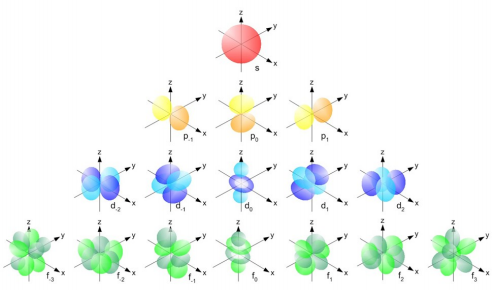Each principal level with n =3 or greater has five d orbitals (ml = -2, -1, 0, +1, +2)

4 of the orbitals have a clover shape with four lobes electron density  around the nucleus and two perpendicular nodal planes

The other orbital (d0) has two lobes on the z -axis and a donut shaped  ring on the xy plane

f Orbitals (1 = 3)

Each principal level with n =4 or greater has seven f orbitals (ml = -3,  -2 -1, 0, +1, +2, +3)

f orbitals have more lobes and nodes than orbitals

○ atomic transitions

A change of an electron from one quantum state to another within an  atom. Allowed when the change in l (angular momentum quantum number) equals  +1 or -1. If that is not the case, the transitions are “forbidden” (occur with much  smaller probability).

○ relationship between # of nodes and n quantum number

A node is a point where the electron probability is zero. The number of  nodes equals the n quantum number minus 1.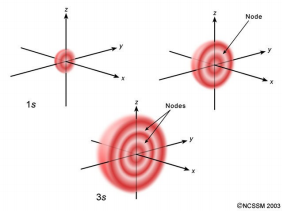○ relationship between average radius of orbital and n quantum number

The different values of n give the average distance of the electron from the nucleus (average radius).

Memorize these!!

∙ The behavior of light: E = hν and ν = c/ λ

∙ the de Broglie relation: λ = h/(mv)

∙ density = mass/volume

∙ Avogadro’s number = 6.022 ∙ 1023

∙ 1 cm3 = 1 mL

Page ExpiredIt looks like your free minutes have expired! Lucky for you we have all the content you need, just sign up here
References: